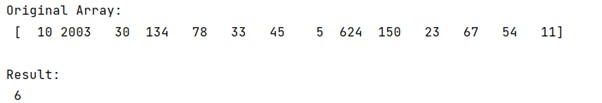# How to count values in a certain range in a NumPy array?

Learn, how to count values in a certain range in a NumPy array?
Submitted by Pranit Sharma, on February 26, 2023

NumPy is an abbreviated form of Numerical Python. It is used for different types of scientific operations in python. Numpy is a vast library in python which is used for almost every kind of scientific or mathematical operation. It is itself an array which is a collection of various methods and functions for processing the arrays.

## Counting values in a certain range in a NumPy array

Suppose that we are given a numpy array with some numerical values. We need to count how many of these values lie in a specific range. For example, we need to count the number of elements that lies between 30 to 150.

NumPy has a counter but it is valid for specific values and not a range of values. Also, if we try the range() function, it will return all the values in a specific range bit, not the count.

A very fine solution for this problem is to use a direct expression stating a condition applied on our array that (30 < arr < 150) on which the sum() function can be applied.

This expression results in a Boolean array with the same shape as arr with the value True for all elements that satisfy the condition. Summing over this Boolean array treats True values as 1 and False values as 0.

Let us understand with the help of an example,

## Python code to count values in a certain range in a NumPy array

```# Import numpy
import numpy as np

# Creating a numpy array
arr = np.array([10,2003,30,134,78,33,45,5,624,150,23,67,54,11])

# Display original array
print("Original Array:\n",arr,"\n")

# Counting all the values lies in a specific range
res = ((30 < arr) & (arr < 150)).sum()

# Display result
print("Result:\n",res)
```

Output:Languages: » C » C++ » C++ STL » Java » Data Structure » C#.Net » Android » Kotlin » SQL
Web Technologies: » PHP » Python » JavaScript » CSS » Ajax » Node.js » Web programming/HTML
Solved programs: » C » C++ » DS » Java » C#
Aptitude que. & ans.: » C » C++ » Java » DBMS
Interview que. & ans.: » C » Embedded C » Java » SEO » HR
CS Subjects: » CS Basics » O.S. » Networks » DBMS » Embedded Systems » Cloud Computing
» Machine learning » CS Organizations » Linux » DOS
More: » Articles » Puzzles » News/Updates